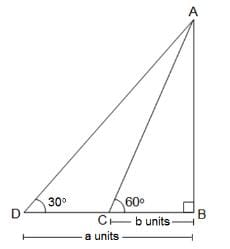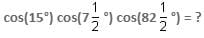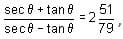CAT  >  Practice Questions Level 2: Trigonometry - 1

# Practice Questions Level 2: Trigonometry - 1 - Notes | Study Level-wise Practice Questions for CAT Preparation - CAT

 1 Crore+ students have signed up on EduRev. Have you?

This EduRev document offers 10 Multiple Choice Questions (MCQs) from the topic Trigonometry (Level - 2). These questions are of Level - 2 difficulty and will assist you in the preparation of CAT & other MBA exams. You can practice/attempt these CAT Multiple Choice Questions (MCQs) and check the explanations for a better understanding of the topic.

Question for Practice Questions Level 2: Trigonometry - 1
Try yourself:Find the value of (cosθ cos(90 - θ) secθ) ÷ (tanθ sin (90 - θ)).

Question for Practice Questions Level 2: Trigonometry - 1
Try yourself:If a = x sinθ + y cosθ and b = y sinθ - x cosθ, then y2 - a2 is equal to

Question for Practice Questions Level 2: Trigonometry - 1
Try yourself:If sin (π cos x) = cos (π sin x), then x equals

Question for Practice Questions Level 2: Trigonometry - 1
Try yourself:In the given figure, AB is the height of a tree. C and D are the two points in a straight line on the ground at b units and a units respectively away from the tree. What is the height of the tree (AB)?Question for Practice Questions Level 2: Trigonometry - 1
Try yourself:A man on the top of a tower observes a car moving towards the base of the tower at an angle of depression α. Ten minutes later, the angle of depression of the car is found to be β. If the tangent of angle α. is equal to 1/√5, co-tangent of angle β is equal to 1/√5 and the car is moving with a uniform speed, find the total time taken by the car to reach the base of the tower.

Question for Practice Questions Level 2: Trigonometry - 1
Try yourself:Question for Practice Questions Level 2: Trigonometry - 1
Try yourself:Ifthen the value of sin is

Question for Practice Questions Level 2: Trigonometry - 1
Try yourself:The angles of elevation of the top of the tower, as observed from each of the points A, B and C on the ground, form a triangle at the same angle α. If R is the circum-radius of the triangle ABC, then the height of the tower is

Question for Practice Questions Level 2: Trigonometry - 1
Try yourself:If sin x + cos y = a and cos x + sin y = b, then what will be the value of tan (x - y)/2 ?

Question for Practice Questions Level 2: Trigonometry - 1
Try yourself:A vertical building and a tower are on the same ground level. From the top of the building, the angle of elevation of the top of the tower is 45° and the angle of depression of the foot of the tower is 60°. Find the height of the tower, if the height of the building is 30 m.

The document Practice Questions Level 2: Trigonometry - 1 - Notes | Study Level-wise Practice Questions for CAT Preparation - CAT is a part of the CAT Course Level-wise Practice Questions for CAT Preparation.
All you need of CAT at this link: CAT

## Level-wise Practice Questions for CAT Preparation

277 docs
 Use Code STAYHOME200 and get INR 200 additional OFF

## Level-wise Practice Questions for CAT Preparation

277 docs

### How to Prepare for CAT

Read our guide to prepare for CAT which is created by Toppers & the best Teachers

Track your progress, build streaks, highlight & save important lessons and more!

,

,

,

,

,

,

,

,

,

,

,

,

,

,

,

,

,

,

,

,

,

;